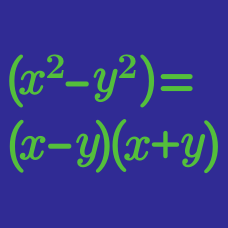Algebra

# Polynomial Factoring: Level 2 Challenges

$\large \sqrt a + b = 7 \\ \large \sqrt b + a = 11$

If $a$ and $b$ are real numbers that satisfy the equation above, what is the value of $a$ and $b$ respectively?

Given that $6y^2=2014$, find the value of

$\frac{9\big(y^4+6y^3+9y^2\big)}{y^2+6y+9}.$

$\large a^3 + b^3 = 2593080, \ \ a + b = 210, \ \ \ \ \ ab = \ ?$

Given that $a+b=1$ and $a^2+b^2=2$, what is the value of $a^7+b^7$?

If $x^2 - 5x - 1 = 0$, then find the value of $x^2 + \frac{1}{x^2}.$

×